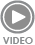# Steps to calculate a gear ratio

Once of the key concepts for their adequate use is the gear ratio (rt). Correctly selecting the gear ratio is essential

The use of gears in a myriad of machines and devices nowadays makes calls for them to be used at their top efficiency to make the most out of their advantages.

Once of the key concepts for their adequate use is the gear ratio (rt). Correctly selecting the gear ratio is essential so that:

The gear can withstand a greater torque, minimizing errors

Motion can be generated in a way that the motor gear torque overcomes the gear’s inertia.

Below we will get to know the required formulas to calculate the gear ratio, and what it is that you should keep in mind for this calculation.

The gar ratio is essential for motion to take place and for the gear to be able to withstand the torque without leading to errors

What is motion transmission?

The gear ratio in a transmission is the ratio between the rotational speeds of two meshing gears.

Since each gear has a different diameter, each of the axes rotates at a different speed when they are both engaged. Modifying the gear ratio is the equivalent of modifying the torque that is applied.

The gear ratio is calculated by dividing the output speed by the input speed (i= Ws/ We) or by dividing the number of teeth of the driving gear by the number of teeth of the driven gear (i= Ze/ Zs).

i = Ws/We

i = Ze/Zs

Geared transmission offer a series of advantages when compared to other types of transmissions. First, they offer a high performance when transmitting forces and motions, with a long service life and high reliability.

But what makes them stand out is the stupendous accuracy of their gear ratio, which means that they can be employed in precision machinery.

Gear ratios in geared transmissions is very precise, which makes these transmissions perfect for precision machinery

Unlike other mechanisms, such as chains or pulleys, their size is small, which means that they can be installed both in both small and large machines and spaces, as well as in places that are hard to access.

Furthermore, their simple maintenance makes geared transmissions one of the most widespread systems in major industries such as automotive.

Parameters to design a geared transmission

When designing a geared transmission, one needs to keep in mind the following parameters:

Law of gearing

The law of gearing defines the situation by which the point of contact between two teeth will always cross point O, when its profile allows it.

This point O must be located in a line that joins both the center of rotation of one of the gears and the center of rotation of the other.

In addition, the pitch radii and the distances between point O and the corresponding centers must coincide.

Gear ratio

The gear ratio (rt) is the ratio between the rotational speeds of two mating gears.

More specifically, it is the quotient of the input and output speeds (rt = ωs / ωe).

The system may cause a reduction if the gear ratio is less than 1, or a multiplication if it is greater than 1.

You might be interested in: Variable speed drives: all you need to know about speed reducers

Contact Ratio

The contact ratio (ε) measures the average number of teeth in contact at all times.

The best contact ratio must be larger than 1.2 to ensure the ability to transmit high loads, offer rigidity to the transmission and allow for a silent and uniform operation.

How to calculate the gear ratio

Calculating a gear ratio can be accomplished in a number of ways. For example:

Calculating the gear ratio of bevel gears

When referring to bevel gears, we need to consider that it will be equivalent to the number of teeth of the driving gear divided by the number of teeth of the driven gear (RT= Z1 / Z2).

You might be interested in: Gear calculation: improve the efficiency of your transmissions.

Calculating the gear ratio of a gear train

In the case of a gear train with two gears, we need to do the following steps:

Count the number of teeth. First, we will need to count the number of teeth of the driving gear and the number of teeth of the driven gear.

Divide the number of teeth of the driven gear by the number of teeth of the driving gear. The resulting number will be the times that the driven, smaller gear will have to turn for the large one to complete one full rotation.

The gear ratio will equal the number of teeth of the driving gear divided by the number of teeth of the driven gear.

In the case of a gear train with more than two gears, we will need to do the following steps:

Identify which is the driving gear and which is the driven gear and divide the number of teeth of the former and the latter. The intermediate gear will not affect the gear ratio of the gear train in the least.

Follow the same steps as you would to find out the gear ratio of a two-gear gear train.

There are many ways to calculate a gear ratio, depending on whether the gears are bevel gears or gear trains with two or more gears, etc.

Calculating the gear ratio of gears and chain

This type of transmission is formed by two gears and a chain of articulated links. In it, the gears rotate in the same direction.

It is used to transmit a motion between distant parallel shafts.

The gear ratio is the result of dividing the number of teeth of the driver gear and the number of teeth of the driven gear.